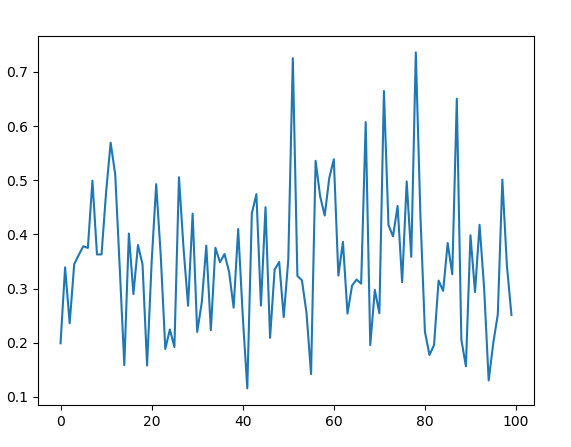Open in App
Not now

# random.betavariate() method in Python

• Last Updated : 17 May, 2020

`betavariate()` is an inbuilt method of the `random` module. It is used to return a random floating point number with beta distribution. The returned value is between 0 and 1.

Syntax : random.betavariate(alpha, beta)

Parameters :
alpha : greater than 0
beta : greater than 0

Returns : a random beta distribution floating number between 0 and 1

Example 1:

 `# import the random module``import` `random`` ` `# determining the values of the parameters``alpha ``=` `5``beta ``=` `10`` ` `# using the betavariate() method``print``(random.betavariate(alpha, beta))`

Output :

`0.5148685287422776`

Example 2: We can generate the number multiple times and plot a graph to observe the beta distribution.

 `# import the required libraries``import` `random``import` `matplotlib.pyplot as plt`` ` ` ` `# store the random numbers in a ``# list``nums ``=` `[]``low ``=` `10``high ``=` `100``mode ``=` `20`` ` `for` `i ``in` `range``(``100``):``    ``temp ``=` `random.betavariate(``5``, ``10``)``    ``nums.append(temp)``     ` `# plotting a graph``plt.plot(nums)``plt.show()`

Output :My Personal Notes arrow_drop_up